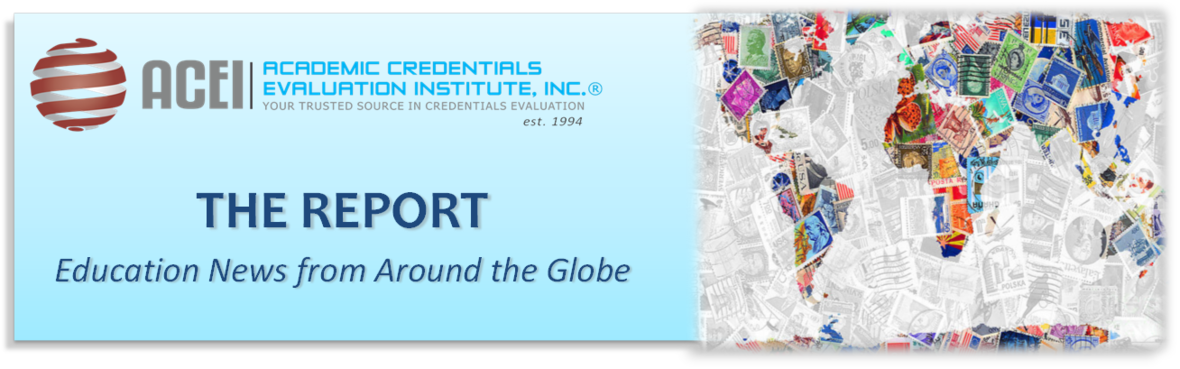# August 2018 - Beverly Hills, CA

ACADEMIC CREDENTIALS EVALUATION INSTITUTE, INC. (ACEI)
Is incorporated in the state of California (1994), and is dedicated to the advancement of international academic exchange and understanding through the dissemination of information on international academic credentials.

 table div table+table+table div table{width:100%;padding:0}table div table+table+table div table img{width:96.23%;padding:0;float:none}table div table+table+table div table td{width:100%;padding:0 1.88% 18px}/* styles */# News Around the worldPHOTO: Australian Party seantor Fraser Anning (ABC News: Adam Kennedy)

# Would Reducing International Student Visas Create University Places for Australians?

Australian Party senator Fraser Anning sees migration as a “critical problem” facing the nation, and among the solution proposed in his controversial first speech to Parliament was a big reduction in student visas to create more places for domestic students. Australian Broadcasting Corporation (ABC) Fact Check takes a look at the evidence and find the Senator’s claims as ill informed. To read more, click here.

 table div table+table+table+table+table+table div table{width:100%;padding:0}table div table+table+table+table+table+table div table img{width:96.23%;padding:0;float:none}table div table+table+table+table+table+table div table td{width:100%;padding:0 1.88% 18px}/* styles */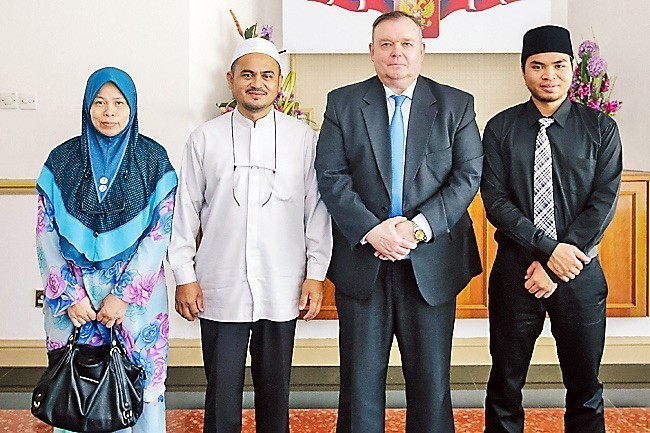Ambassador of the Russian Federation to Brunei Darussalam Vladlen Semivolos with Muhammad Miqdam bin Zaini and his parents. – BAHYIAH BAKIR

# Forging Partnerships and Exchanges in Higher Education

The Ministries of Education of Brunei and Russia are currently working on a Memorandum of Understanding (MOU) on cooperation in higher education and science, which will become an umbrella agreement contributing to the development of exchanges and partnerships between institutions of higher learning in the two countries. To read more, click here

 table div table+table+table+table+table+table+table+table+table div table{width:100%;padding:0}table div table+table+table+table+table+table+table+table+table div table img{width:96.23%;padding:0;float:none}table div table+table+table+table+table+table+table+table+table div table td{width:100%;padding:0 1.88% 18px}/* styles */Image Credit: Alexander Glandien

# The Tweet that Sparked a Feud

If you’re wondering why Saudi Arabia expelled the Canadian Ambassador for the country and has withdrawn its 15,000 students from Canadian colleges and universities, check out ACEI’s blog for a quick summary. To read more, click here.

 table div table+table+table+table+table+table+table+table+table+table+table div table{width:100%;padding:0}table div table+table+table+table+table+table+table+table+table+table+table div table img{width:96.23%;padding:0;float:none}table div table+table+table+table+table+table+table+table+table+table+table div table td{width:100%;padding:0 1.88% 18px}/* styles */# Strengthening Regulation of Extracurricular Education Institutions

China will strengthen regulation of institutions providing extracurricular classes or off-campus training, according to a recent guideline issued by the State Council General Office. To read more, click here.

 table div table+table+table+table+table+table+table+table+table+table+table+table+table div table{width:100%;padding:0}table div table+table+table+table+table+table+table+table+table+table+table+table+table div table img{width:96.23%;padding:0;float:none}table div table+table+table+table+table+table+table+table+table+table+table+table+table div table td{width:100%;padding:0 1.88% 18px}/* styles */# How China’s Middle-Class Moms Become Their Kids’ Agents

"Shadow education” — extracurricular classes that reinforce existing school curricula — is extremely popular among urban, middle-class Chinese parents. Last year, the after-school tutoring market for primary and secondary school students was worth 624 billion yuan (\$93.2 billion), according to an influential industry report. And most companies are targeting one consumer group: moms. To read more, click here.

 table div table+table+table+table+table+table+table+table+table+table+table+table+table+table+table div table{width:100%;padding:0}table div table+table+table+table+table+table+table+table+table+table+table+table+table+table+table div table img{width:96.23%;padding:0;float:none}table div table+table+table+table+table+table+table+table+table+table+table+table+table+table+table div table td{width:100%;padding:0 1.88% 18px}/* styles */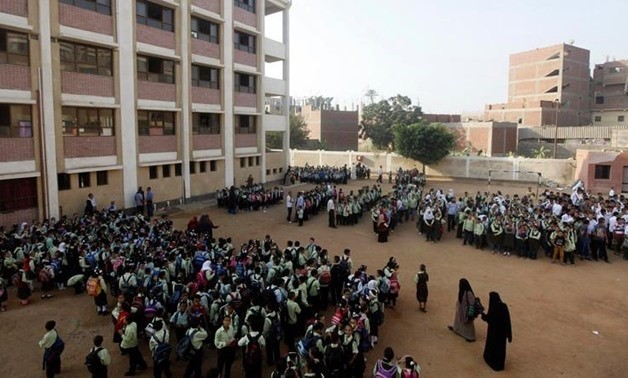Students line up on the first day of their new school year at a government school in Giza – Reuter

# Plans to Establish 1000 New Schools to Reduce Class Size

On August 20, 2018, the Minister of Finance Mohamed Moeit said that the government will work on completing procedures required to establish a project aiming to establish 1,000 language schools, following the Eid al-Adha ends on August 24, 2018. To read more, click here.

 table div table+table+table+table+table+table+table+table+table+table+table+table+table+table+table+table+table+table div table{width:100%;padding:0}table div table+table+table+table+table+table+table+table+table+table+table+table+table+table+table+table+table+table div table img{width:96.23%;padding:0;float:none}table div table+table+table+table+table+table+table+table+table+table+table+table+table+table+table+table+table+table div table td{width:100%;padding:0 1.88% 18px}/* styles */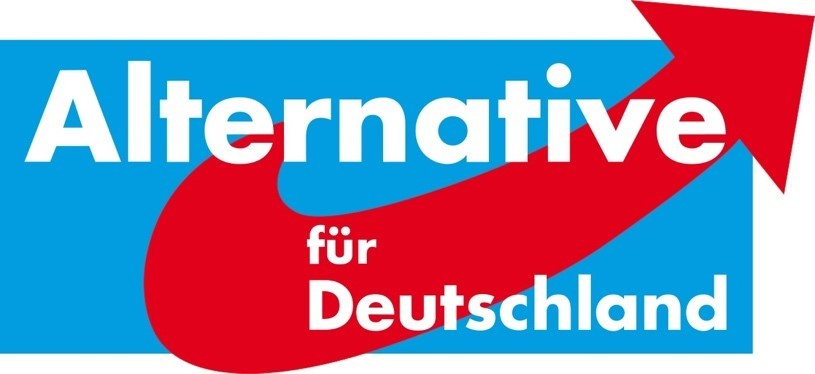# Far Right Turns its Ire on German Universities

Using tropes common in the U.S. and Britain, the Alternative for Germany has accused institutions of being in thrall to the far left. To read more, click here.

 table div table+table+table+table+table+table+table+table+table+table+table+table+table+table+table+table+table+table+table+table div table{width:100%;padding:0}table div table+table+table+table+table+table+table+table+table+table+table+table+table+table+table+table+table+table+table+table div table img{width:96.23%;padding:0;float:none}table div table+table+table+table+table+table+table+table+table+table+table+table+table+table+table+table+table+table+table+table div table td{width:100%;padding:0 1.88% 18px}/* styles */# China Grants Scholarships to Ghanaian Students

In presenting letters of admission the Ghanian students, Mr. Shi Ting Wang, China's Ambassador to Ghana, said "President Nana Addo Dankwa Akufo-Addo attached great importance to education and had invested a large amount of resources to promote the development of education." To read more, click here.

 table div table+table+table+table+table+table+table+table+table+table+table+table+table+table+table+table+table+table+table+table+table+table div table{width:100%;padding:0}table div table+table+table+table+table+table+table+table+table+table+table+table+table+table+table+table+table+table+table+table+table+table div table img{width:96.23%;padding:0;float:none}table div table+table+table+table+table+table+table+table+table+table+table+table+table+table+table+table+table+table+table+table+table+table div table td{width:100%;padding:0 1.88% 18px}/* styles */# Revamp of Regulatory Boards in Trouble

The Indian government's move to replace the higher education regulator, the University Grants Commission (UGC), with a new body to improve quality and allow institutions more autonomy faces opposition from parties in parliament and criticism that it would increase government control and politicization of education. To read more, click here.

 table div table+table+table+table+table+table+table+table+table+table+table+table+table+table+table+table+table+table+table+table+table+table+table+table div table{width:100%;padding:0}table div table+table+table+table+table+table+table+table+table+table+table+table+table+table+table+table+table+table+table+table+table+table+table+table div table img{width:96.23%;padding:0;float:none}table div table+table+table+table+table+table+table+table+table+table+table+table+table+table+table+table+table+table+table+table+table+table+table+table div table td{width:100%;padding:0 1.88% 18px}/* styles */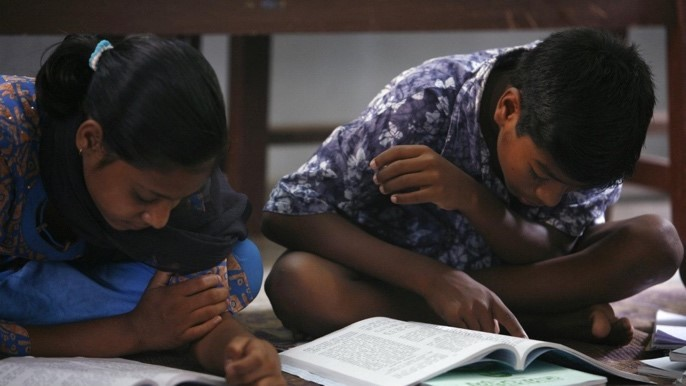Photo Source: QuartzIndia

# Students at India’s Urban Private Schools Struggle with English Proficiency

India’s urban private schools, catering to middle-class families, were for long seen as better off than most institutions in the country’s outdated education system. But a new study on the reading proficiency of students in private, unaided English-medium schools suggests this is hardly the case. To read more, click here.

 table div table+table+table+table+table+table+table+table+table+table+table+table+table+table+table+table+table+table+table+table+table+table+table+table+table+table+table div table{width:100%;padding:0}table div table+table+table+table+table+table+table+table+table+table+table+table+table+table+table+table+table+table+table+table+table+table+table+table+table+table+table div table img{width:96.23%;padding:0;float:none}table div table+table+table+table+table+table+table+table+table+table+table+table+table+table+table+table+table+table+table+table+table+table+table+table+table+table+table div table td{width:100%;padding:0 1.88% 18px}/* styles */# What’s it like to study abroad in Italy?

Interested in studying abroad in Italy? We found a complete guide to the lingo, culture and making friends. To read more, click here

 table div table+table+table+table+table+table+table+table+table+table+table+table+table+table+table+table+table+table+table+table+table+table+table+table+table+table+table+table+table+table div table{width:100%;padding:0}table div table+table+table+table+table+table+table+table+table+table+table+table+table+table+table+table+table+table+table+table+table+table+table+table+table+table+table+table+table+table div table img{width:96.23%;padding:0;float:none}table div table+table+table+table+table+table+table+table+table+table+table+table+table+table+table+table+table+table+table+table+table+table+table+table+table+table+table+table+table+table div table td{width:100%;padding:0 1.88% 18px}/* styles */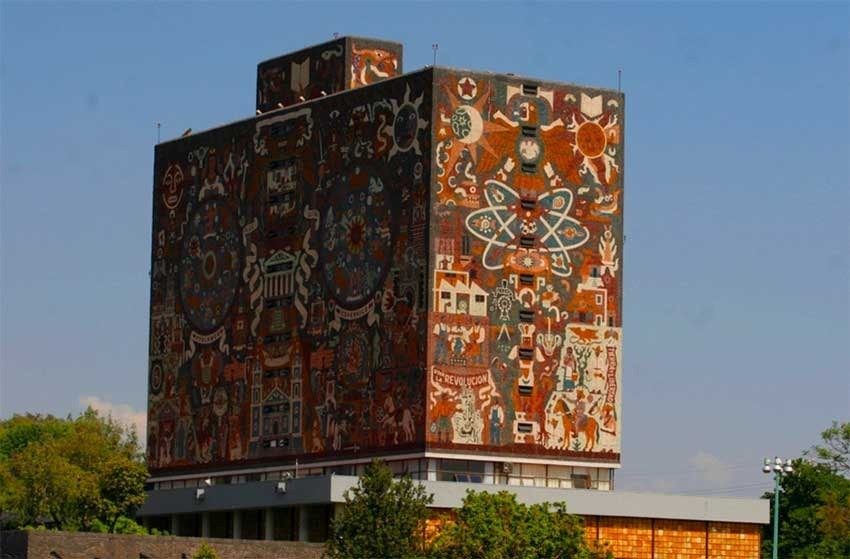Autonomous University of Mexico (Photo Source: Mexico Daily News)

# Number of University Graduates Well Below OECD Average

Recent statistics show that Mexican adults are less likely to have a university degree than citizens of any other OECD country. To read more, click here.

 table div table+table+table+table+table+table+table+table+table+table+table+table+table+table+table+table+table+table+table+table+table+table+table+table+table+table+table+table+table+table+table+table+table div table{width:100%;padding:0}table div table+table+table+table+table+table+table+table+table+table+table+table+table+table+table+table+table+table+table+table+table+table+table+table+table+table+table+table+table+table+table+table+table div table img{width:96.23%;padding:0;float:none}table div table+table+table+table+table+table+table+table+table+table+table+table+table+table+table+table+table+table+table+table+table+table+table+table+table+table+table+table+table+table+table+table+table div table td{width:100%;padding:0 1.88% 18px}/* styles */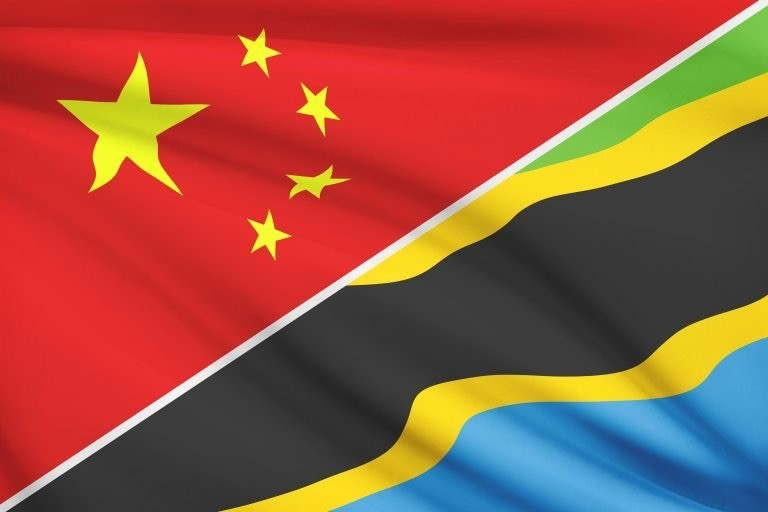# Chinese Scholarship Program for Tanzanian Students

The government of Tanzania has appealed to the Chinese government to increase the number of Tanzanian students benefiting from the ongoing Chinese scholarship programs. To read more, click here

 table div table+table+table+table+table+table+table+table+table+table+table+table+table+table+table+table+table+table+table+table+table+table+table+table+table+table+table+table+table+table+table+table+table+table+table div table{width:100%;padding:0}table div table+table+table+table+table+table+table+table+table+table+table+table+table+table+table+table+table+table+table+table+table+table+table+table+table+table+table+table+table+table+table+table+table+table+table div table img{width:96.23%;padding:0;float:none}table div table+table+table+table+table+table+table+table+table+table+table+table+table+table+table+table+table+table+table+table+table+table+table+table+table+table+table+table+table+table+table+table+table+table+table div table td{width:100%;padding:0 1.88% 18px}/* styles */Photo: iStock

# Will Artificial Intelligence Make Education Relevant?

Digital Learning solutions equipped with artificial intelligence are making revolutionary changes to the way education is imparted in students with varied interests and capabilities. To read more, click here.

 table div table+table+table+table+table+table+table+table+table+table+table+table+table+table+table+table+table+table+table+table+table+table+table+table+table+table+table+table+table+table+table+table+table+table+table+table+table+table div table{width:100%;padding:0}table div table+table+table+table+table+table+table+table+table+table+table+table+table+table+table+table+table+table+table+table+table+table+table+table+table+table+table+table+table+table+table+table+table+table+table+table+table+table div table img{width:96.23%;padding:0;float:none}table div table+table+table+table+table+table+table+table+table+table+table+table+table+table+table+table+table+table+table+table+table+table+table+table+table+table+table+table+table+table+table+table+table+table+table+table+table+table div table td{width:100%;padding:0 1.88% 18px}/* styles */table.module-39{width:84.72%;padding:0}table div table+table+table+table+table+table+table+table+table+table+table+table+table+table+table+table+table+table+table+table+table+table+table+table+table+table+table+table+table+table+table+table+table+table+table+table+table+table+table+table div table{width:84.72%;float:none;margin-left:auto;margin-right:auto;padding:0}table div table+table+table+table+table+table+table+table+table+table+table+table+table+table+table+table+table+table+table+table+table+table+table+table+table+table+table+table+table+table+table+table+table+table+table+table+table+table+table+table div table a{border:0 none;text-decoration:none}table div table+table+table+table+table+table+table+table+table+table+table+table+table+table+table+table+table+table+table+table+table+table+table+table+table+table+table+table+table+table+table+table+table+table+table+table+table+table+table+table div table img{width:100%!important;border:0 none;text-decoration:none}table div table+table+table+table+table+table+table+table+table+table+table+table+table+table+table+table+table+table+table+table+table+table+table+table+table+table+table+table+table+table+table+table+table+table+table+table+table+table+table+table div table td{width:100%;padding:0}/* styles */

# Digital Delivery of Evaluation Reports: SecurePathway©:

ACEI is a proud signatory of the Groningen Declaration Network which “seeks common ground in best serving the academic and professional mobility needs of citizens worldwide by bringing together key stakeholders in the Digital Student Data Ecosystem.” For the past two years, ACEI has committed itself to this shared mission through the introduction of SecurePathway©, an ACEI proprietary digital platform for the secure delivery of official evaluation reports and supporting educational credentials. For information on ACEI’s SecurePathway©.

Signing up with ACEI’s SecurePathway©, is free and does not cost the institutions any fees. To receive ACEI expert reports and certified academic credentials via SecurePathway©, complete this form.

*SecurePathway Registration Form*/* styles */ ACEI’s Advisory Services provide institutions and organizations looking at expanding their international student recruitment with customized solutions to help overcome enrollment challenges, uncover opportunities, and devise an innovative and holistic approach to international enrollment. For more information on ACEI’s Advisory Services and how we can assist you, please contact Yolinisse Moreno, Director of Programs & Communications at ymoreno@acei-global.org.
 table div table+table+table+table+table+table+table+table+table+table+table+table+table+table+table+table+table+table+table+table+table+table+table+table+table+table+table+table+table+table+table+table+table+table+table+table+table+table+table+table+table+table+table+table div table{width:100%;padding:0}table div table+table+table+table+table+table+table+table+table+table+table+table+table+table+table+table+table+table+table+table+table+table+table+table+table+table+table+table+table+table+table+table+table+table+table+table+table+table+table+table+table+table+table+table div table img{width:96.23%;padding:0;float:none}table div table+table+table+table+table+table+table+table+table+table+table+table+table+table+table+table+table+table+table+table+table+table+table+table+table+table+table+table+table+table+table+table+table+table+table+table+table+table+table+table+table+table+table+table div table td{width:100%;padding:0 1.88% 18px}/* styles *//* styles */ ACEI offers a comprehensive range of professional services that include: international credential evaluations; translations; consulting; and training. For more information on ACEI, please visit www.acei-global.org or contact: Tel: 1-310-275-3530; Email: acei@acei-global.org. ACEI was founded and incorporated in 1994 in the State of California and is a Charter and Endorsed Member of the Association of International Credential Evaluators (AICE).
 Like   Tweet   Pin   +1   in